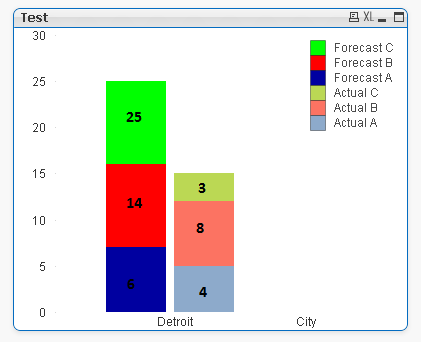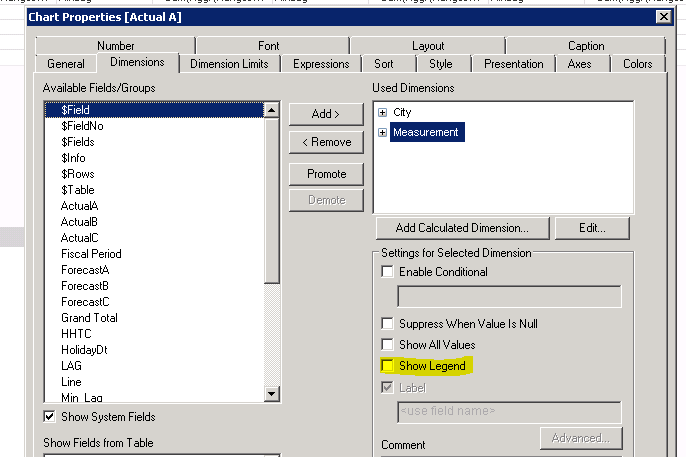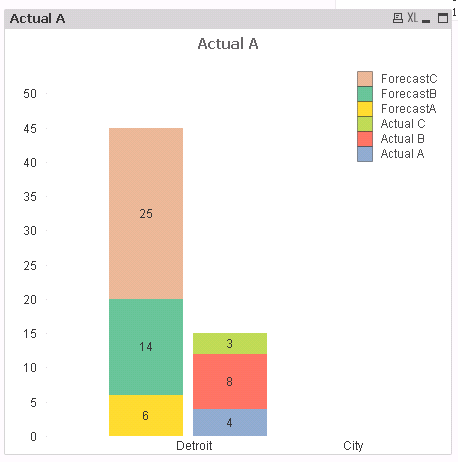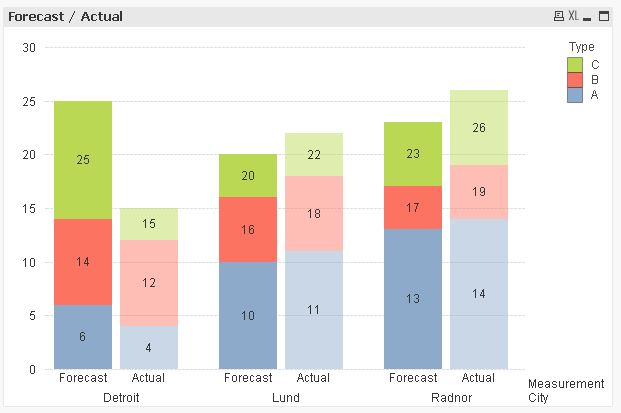# QlikView App Development

Discussion Board for collaboration related to QlikView App Development.

Announcements
The #1 reason QlikView customers adopt Qlik Sense is a desire for a modern BI experience. Read More
cancel
Showing results for
Did you mean:
HighlightedContributor

## Is there anyway I can replicate this chart in QlikView?

I am struggling to create this chart in Qlik. I can create the bars by expressions like Forecast C = Forecast C- Forecast B- Forecast A. but how can I show the Actual values?Tags (4)
6 Replies
HighlightedChampion III

## Re: Is there anyway I can replicate this chart in QlikView?

Can you share your sample file with some data to look into?

HighlightedContributor

## Re: Is there anyway I can replicate this chart in QlikView?

Actually, I need to create a graph like this so I don't have any qvw file but I can share the script for the bars.

City, ForecastA, ForecastB, ForecastC, Measurement

Detroit, 6, 14, 25, Forecast

];

City, ActualA, ActualB, ActualC, Measurement

Detroit, 4, 12, 15, Actual

];

Forecast bar is what I want to do and Actual bar shows what I can accomplish.

HighlightedChampion III

## Re: Is there anyway I can replicate this chart in QlikView?

Try this?

City:

City, ForecastA, ForecastB, ForecastC, Measurement

Detroit, 6, 14, 25, Forecast

];

Concatenate

City, ActualA, ActualB, ActualC, Measurement

Detroit, 4, 12, 15, Actual

];

Dim: like below image

For measurement dimension uncheck Show Legend.= Sum(ActualA)

= Sum(ActualB)-Sum(ActualA)

=Sum(ActualC) -Sum(ActualB)

= Sum(ForecastA)

=Sum(ForecastB)

=Sum(ForecastC)

Below is what you getHighlightedContributor

## Re: Is there anyway I can replicate this chart in QlikView?

Yes. I can replicate that but what I want is to 25 bar as 25-14-6 = 5 height, 14 bar as 8 respectively. but the number inside the bar should show 25,14,6. Let me know if you do not understand my question. Thank you.

HighlightedChampion III

## Re: Is there anyway I can replicate this chart in QlikView?

try below for Forecast expressions:

= Sum(ForecastA)

= Dual(Sum(ForecastB), Sum(ForecastB) - Sum(ForecastA))

=Dual(Sum(ForecastC), Sum(ForecastC) - Sum(ForecastB) - Sum(ForecastA))HighlightedMVP

## Re: Is there anyway I can replicate this chart in QlikView?

Hi,

maybe one solution could be:Dual(Sum(Value),RangeSum(Sum(Value),-Above(Sum(Value))))Color(RowNo()) bitand ARGB(Pick(Match(Measurement,'Actual','Forecast'),120,255),255,255,255)

```tabValues:
CrossTable (Type, Value, 2)
City, Measurement, A, B, C
Detroit, Forecast, 6, 14, 25
Lund, Forecast, 10, 16, 20
];

CrossTable (Type, Value, 2)